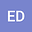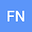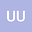loading page

$\mathcal{I}$-Convergent Functions Defined On Amenable Semigroups
•••Erdinç Dündar
Afyon Kocatepe Universitesi
Author ProfileUğur Ulusu
Sivas Cumhuriyet Universitesi
Author Profile## Abstract

In this paper we firstly introduce and study the concepts of \linebreak $\mathcal{I}$-convergence, $\mathcal{I}^*$-convergence, $\mathcal{I}$-Cauchy sequence and $\mathcal{I}^*$-Cauchy sequence of functions defined on discrete countable amenable groups, where $\mathcal{I}$ is an ideal of subsets of the amenable semigroup $G$. Secondly, we introduce and examine $\mathcal{I}$-limit points and $\mathcal{I}$-Cluster points of functions defined on discrete countable amenable groups. Finally, we introduce and investigate $\mathcal{I}$-limit superior and $\mathcal{I}$-limit inferior of functions defined on discrete countable amenable groups.